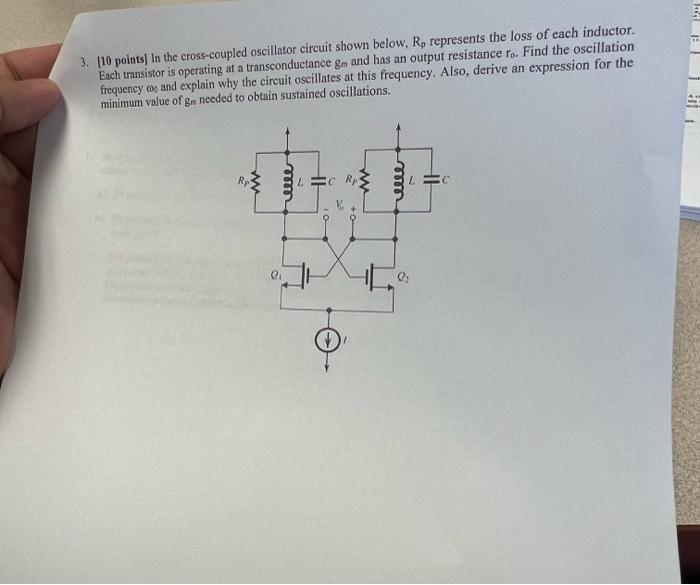# Question 3. [10 points in the cross-coupled oscillator circuit shown below, Ro represents the loss of each inductor. Each transistor is operating at a transconductance gm and has an output resistance ro. Find the oscillation frequency cop and explain why the circuit oscillates at this frequency. Also, derive an expression for the minimum value of ge needed to obtain sustained oscillations. - w mm 11 C فففف LC w +0 0 2 5NCH2IV The Asker · Electrical EngineeringTranscribed Image Text: 3. [10 points in the cross-coupled oscillator circuit shown below, Ro represents the loss of each inductor. Each transistor is operating at a transconductance gm and has an output resistance ro. Find the oscillation frequency cop and explain why the circuit oscillates at this frequency. Also, derive an expression for the minimum value of ge needed to obtain sustained oscillations. - w mm 11 C فففف LC w +0 0 2 5
More
Transcribed Image Text: 3. [10 points in the cross-coupled oscillator circuit shown below, Ro represents the loss of each inductor. Each transistor is operating at a transconductance gm and has an output resistance ro. Find the oscillation frequency cop and explain why the circuit oscillates at this frequency. Also, derive an expression for the minimum value of ge needed to obtain sustained oscillations. - w mm 11 C فففف LC w +0 0 2 5